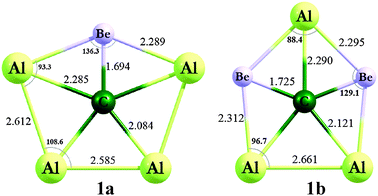# Are there any compounds with pentavalent carbon?

##### 1 Answer
Apr 20, 2015

Scientists first prepared methanium ion in 1950.

#### Explanation:

Methanium ion

Fluoroantimonic acid ${\text{HSbF}}_{6}$ reacts with methane to form methanium ion, $\text{CH"_5^"+}$.

It is a fluxional ion, in which the $\text{C-H}$ bonds are continually breaking and re-forming even at 4 K.In the animation above, the black ball in the middle is the carbon atom, and the red and white ones are hydrogen atoms. The blue clouds represent the electron pairs.

A theoretical pentacoordinate carbon

Theoretical chemists have predicted that ${\text{CBe}}_{5}^{4 -}$ would have a planar pentacoordinate carbon and might even be stable in the gas phase.The bonds are not ordinary σ bonds. Instead, they involve various σ and π orbitals involving the whole molecule.

$\text{CAl"_4"Be}$ and ${\text{CAl"_3"Be}}_{2}^{-}$ are also theoretical possibilities.Heptacoordinate carbon?

Another theoretical possibility is ${\text{CTi}}_{7}^{2 +}$, with a $\text{C}$ atom at the centre of a pentagonal bipyramid — a heptacoordinate $\text{C}$ atom!